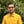Trusted answers to developer questions
Trusted Answers to Developer Questions

Related Tags

ruby
math
hypotenuse
communitycreator

# What is hypot() in Ruby?Harris Amjad

Grokking Modern System Design Interview for Engineers & Managers

Ace your System Design Interview and take your career to the next level. Learn to handle the design of applications like Netflix, Quora, Facebook, Uber, and many more in a 45-min interview. Learn the RESHADED framework for architecting web-scale applications by determining requirements, constraints, and assumptions before diving into a step-by-step design process.

The hypot() function in Ruby returns the hypotenuse of a right-angled triangle from its length and base, which are sent as parameters.

The mathematical formula to calculate the hypotenuse from length and base is as follows:

hypotenuse = sqrt( length^2 + base^2 )


Figure 1 shows the visual representation of the hypot() function.

Figure 1: Visual representation of hypot() function

This function requires the Math module.

## Syntax

hypot(length, base)


## Parameters

This function requires length and base as parameters.

## Return value

This function returns the hypotenuse of the right-angled triangle from its length and base, which are sent as parameters.

## Example

# the hypotenuse of right angled triangle# length=10# base=10print "The hypotenuse of triangle with length = 10 and base = 10: ",Math.hypot(10,10)," \n"# length=4# base=6print "The hypotenuse of triangle with length = 4 and base = 6: ",Math.hypot(4,6)," \n"

RELATED TAGS

ruby
math
hypotenuse
communitycreator

CONTRIBUTORHarris Amjad

Grokking Modern System Design Interview for Engineers & Managers

Ace your System Design Interview and take your career to the next level. Learn to handle the design of applications like Netflix, Quora, Facebook, Uber, and many more in a 45-min interview. Learn the RESHADED framework for architecting web-scale applications by determining requirements, constraints, and assumptions before diving into a step-by-step design process.

Keep Exploring

Learn in-demand tech skills in half the time